TRIGONOMETRY FOR STATICS

PART 2:

The fundamental relation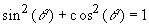suggests that the sine and cosine can be visualized by using a circle of unit radius. To do this, draw a circle of unit radius, as shown in the figure. Next draw a radial line from the center of the circle to the its arc and making a counter clockwise angle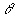with the horizontal axis as shown in the figure. The projection of this line onto the horizontal axis is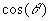, the projection of this line onto the vertical axis is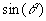, and if the radial line is extended to intersect the vertical line AB one can get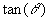as shown in the figure.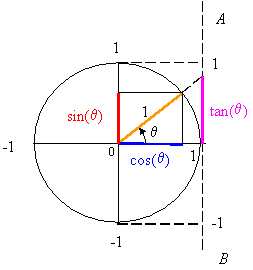From the unit circle one immediately discovers that the sine and cosine functions can have values from -1 to 1, and that the tangent can have any value form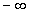to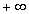.

One denotes the quadrants of the unit circle as shown in the figure. It can be seen that the sine has positive value in the first and second quadrants, and negative value in the third and fourth quadrants. The cosine has positive value in the first and fourth quadrant and negative value in the second and third quadrants. The tangent has positive value in the first and third quadrants and negative value in the second and fourth quadrants.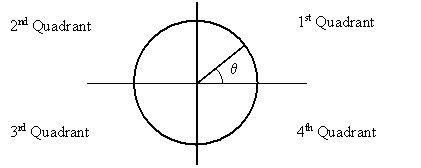The unit circle can also help one memorize the values of the trigonometric functions. For example, at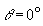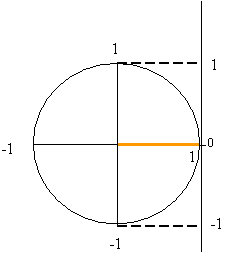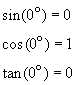At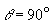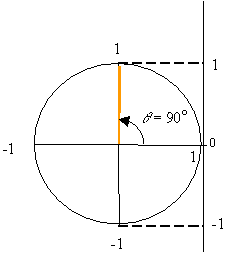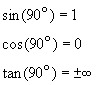At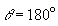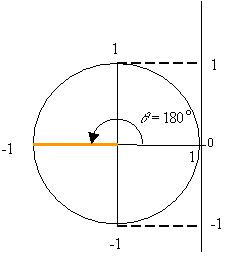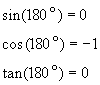At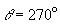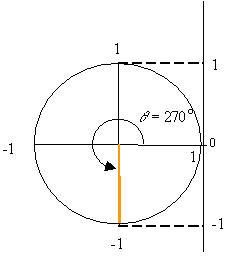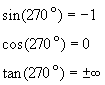The inverse trigonometric functions are: arcsine, arccosine, and arctangent. For a specific value z, these are written as: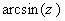,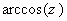,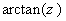. For example, the function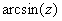provides the angles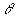that has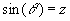. In a similar manner,and, respectively, provide the angles for which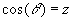and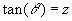.

For example,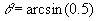means the anglefor which the sine has a value of 0.5. Thus, one solution is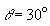. Likewise,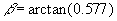has a solution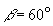.

The inverse trigonometric functions are also written as sin-1, cos-1, and tan-1. For example,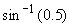is the same as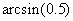. This contradicts the convention established for positive exponents. Therefore, even though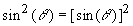The inverse trigonometric functions are multi valued. For example, the angles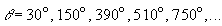all satisfy the relation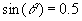and are, therefore, the solutions to. This can clearly be seen on the unit circle since the projection of radial lines at 30o and 150o onto the vertical axis are the same.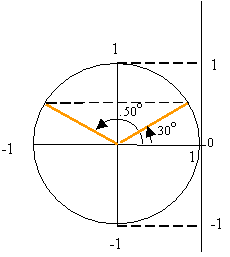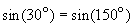On the unit circle the addition of 360o to any angle results in a new radial line that falls on top of the original radial line. Therefore, the value of any trigonometric functions at an angleis the same as its value at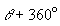. This is also true for the addition of any integer multiple of 360o so that, for example,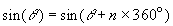for any integer n.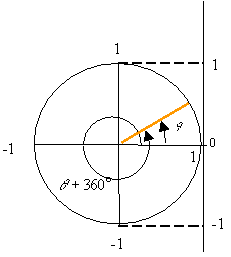The law of sines states that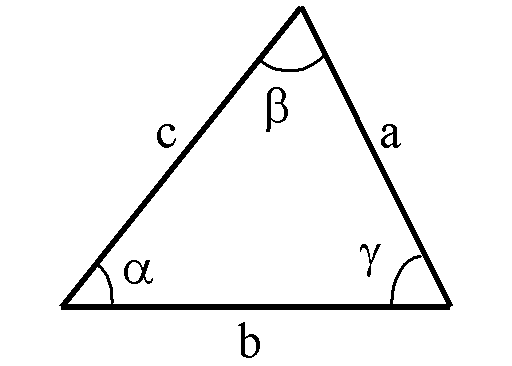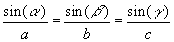This can be shown by considering the triangles AXB and CXB in the following figure. We have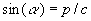and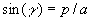, hence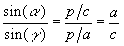or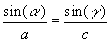. In a similar manner one can show that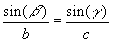.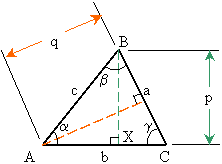The law of cosines states thatThis can be shown by considering the triangle BXC that gives:a2 = p2 + (CX )2 = p2 + (b - AX)2

or a2 = p2 + b2 + (AX)2 - 2b(AX) (1)

Considering the triangle AXB one gets:

p2 + (AX)2 = c2 and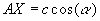Substituting these into (1) one obtains: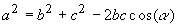The other relations are obtained in a similar manner.

GO TO PART 3

GO BACK TO PART 1